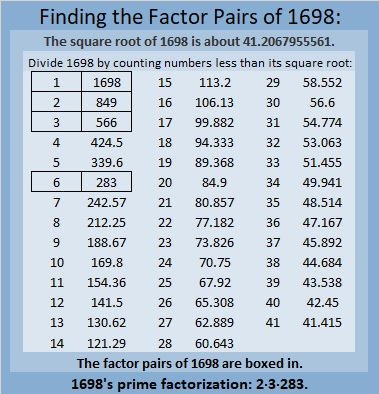# 1698 A Little Virgács and Candy

Contents

### Today’s Puzzle:

If you were a child in Hungary, you might have found some virgács and some candy in your boot this morning. Mikulás (St. Nick) would have given you the candy because of how good you’ve been this year, and the virgács for those times you weren’t so good.

This virgács and candy puzzle is like a mixed-up multiplication table. It is a lot easier to solve because I made it a level 3 puzzle. First, find the common factor of 56 and 72 that will allow only numbers between 1 and 12 to go in the first column. Put the factors in the appropriate cells, then work your way down the puzzle, row by row until each number from 1 to 12 is in both the first column and the top row.### Factors of 1698:

• 1698 is a composite number.
• Prime factorization: 1698 = 2 × 3 × 283.
• 1698 has no exponents greater than 1 in its prime factorization, so √1698 cannot be simplified.
• The exponents in the prime factorization are 1, 1, and 1. Adding one to each exponent and multiplying we get (1 + 1)(1 + 1)(1 + 1) = 2 × 2 × 2 = 8. Therefore 1698 has exactly 8 factors.
• The factors of 1698 are outlined with their factor pair partners in the graphic below.### More About the Number 1698:

1698 = 2(849)(1), so it is a leg in the Pythagorean triple calculated from
2(849)(1), 849² – 1², 849² + 1².

1698 = 2(283)(3), so it is a leg in the Pythagorean triple calculated from
2(283)(3), 283² – 3², 283² + 3².

This site uses Akismet to reduce spam. Learn how your comment data is processed.